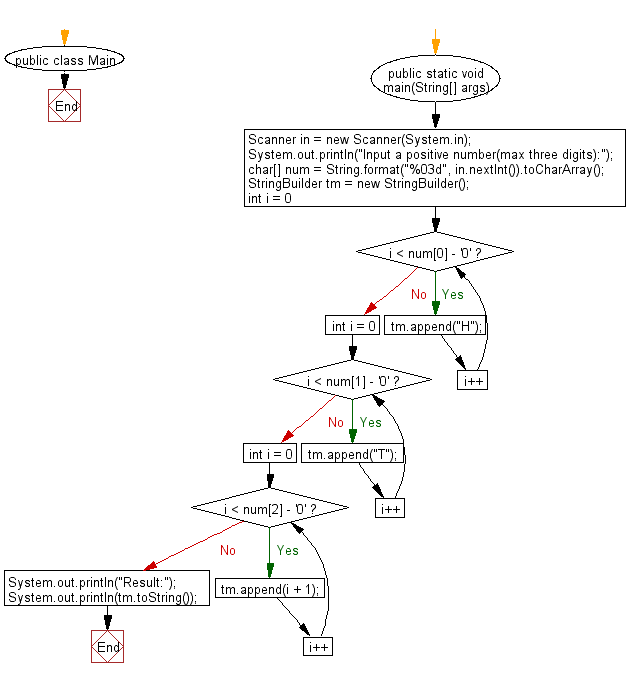# Java Exercises: Convert 3 digits positive number in given format

## Java Basic: Exercise-246 with Solution

Let us use the letter H to mean "hundred", the letter T to mean "ten" and “1, 2, . . . n” to represent the ones digit n (<10).
Write a Java program to convert 3 digits positive number in above format. For example, 234 should be output as BBSSS1234 because it has 2 "hundreds", 3 "ten", and 4 of the ones.

Input:
235
230
Output:
HHTTT12345
HHTTT

Sample Solution:

Java Code:

``````import java.util.Scanner;

public class Main {
public static void main(String[] args) {
Scanner in = new Scanner(System.in);
System.out.println("Input a positive number(max three digits):");
char[] num = String.format("%03d", in.nextInt()).toCharArray();
StringBuilder tm = new StringBuilder();
for (int i = 0; i < num - '0'; i++) {
tm.append("H");
}
for (int i = 0; i < num - '0'; i++) {
tm.append("T");
}
for (int i = 0; i < num - '0'; i++) {
tm.append(i + 1);
}
System.out.println("Result:");
System.out.println(tm.toString());
}
}
```
```

Sample Output:

```Input a positive number(max three digits):
235
Result:
HHTTT12345
```

Pictorial Presentation:Flowchart:Java Code Editor:

What is the difficulty level of this exercise?

﻿

## Java: Tips of the Day

Parsing dates:

```import java.io.*;
import java.util.*;
import java.text.*;

String s = "2001/09/23 14:39";

SimpleDateFormat formatter = new SimpleDateFormat ("yyyy/MM/dd H:mm");
Date d = formatter.parse(s, new ParsePosition(0));
```